# Random Forests for Complete Beginners

Random Forests is one of my favorite data mining algorithms. Invented by Leo Breiman and Adele Cutler back in the last century, it has retained its authenticity up to this day, no changes were added to it since its invention.

Without any exaggeration, it is one of the few universal algorithms. Random forests allow solving both the problems of regression and classification as well. It is good for searching for anomalies and selecting predictors. What is more, this algorithm is technically difficult to apply incorrectly. It is surprisingly simple in its essence. Unlike other algorithms, it has few configurable parameters. And at the same time, it is amazingly accurate.

Wow, so many advantages of using Random forests! It seems like a miracle for machine learning engineers ;) So, if you don’t know yet how it works, it’s the right time to fix this situation. Here is a learning adventure for beginners, where we see things in terms of branches, leaves, and Random forests, of course.

Without further ado, let’s get started!

# Decision Trees in a Nutshell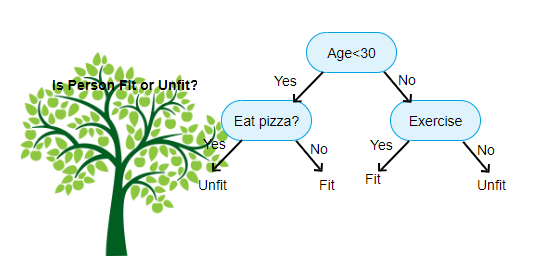Let’s first start with Decision Trees, because logically, there is no forest without trees.

Decision Trees is a non-parametric supervised learning algorithm that builds classification or regression models in the form of a tree structure. It breaks down a data set into smaller and smaller subsets while at the same time an associated decision tree is incrementally developed.

A Random Forest is actually just a bunch of Decision Trees. The goal is to create a model that predicts the value of a target variable by learning simple decision rules inferred from the data features.

A decision tree is a flowchart-like structure in which each internal node represents a “test” on an attribute (e.g. whether a coin flip comes up heads or tails), each branch represents the outcome of the test, and each leaf node represents a class label (decision taken after computing all attributes). The paths from the root to the leaf represent classification rules.

A decision tree consists of three types of nodes:
• Decision nodes — typically represented by squares
• Chance nodes — typically represented by circles
• End nodes — typically represented by triangles

So all-in-all, the learned tree can also be represented as a nested if-else rule to improve human readability. Trees have a high risk of overfitting the training data as well as becoming computationally complex if they are not constrained and regularized properly during the growing stage. This overfitting implies a low bias, high variance trade-off in the model. Therefore, in order to deal with this problem, we use Ensemble Learning, an approach that allows us to correct this overlearning habit and hopefully, arrives at better, stronger results.

# What is an Ensemble Method?

The method of ensembles is based on training algorithms that form many classifiers and then segment new data points, starting from voting or averaging. The original ensemble method is nothing but Bayesian averaging, but later algorithms include output coding error correction, bagging and boosting. Boosting is aimed at turning weak models into strong ones by building an ensemble of classifiers. Bagging also aggregates advanced classifiers, but it uses parallel training of basic classifiers. In the language of mathematical logic, bagging is an improving union, and boosting is an improving intersection.

In our case, a Random Forest (strong learner) is built as an ensemble of Decision Trees (weak learners) to perform different tasks such as regression and classification.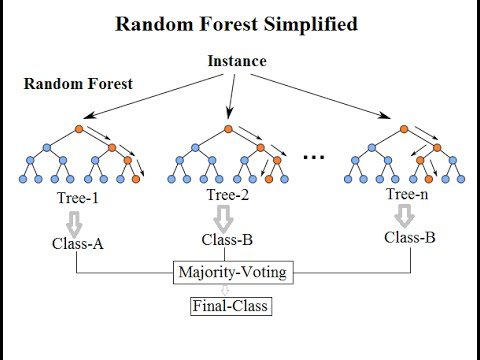# What Is the Idea of Random Forest?

The idea is simple: let’s say we have some very weak algorithm, say, a decision tree. If we make a lot of different models using this weak algorithm and average the result of their predictions, then the final result will be much better. This is the so-called Ensemble learning in action.

Well, here is a reason why Random forest is called this way, cause it creates many decision trees for the data and then averages the result of their predictions. A large number of decision trees are the parameters of the method, each of which is built according to a sample obtained from the original training select using bootstrap (sample with return).

An important point here is the element of randomness in the creation of each tree. After all, it is clear that if we create many identical trees, then the result of their averaging will have the accuracy of one tree.

Simple explanation

A random forest is a collection of random decision trees (the number of n_estimators in sklearn). You need to understand how to create one random decision tree.

Roughly speaking, to build a random decision tree that you start with a subset of your training samples. On each node, you arbitrarily draw a subset of functions (the number is determined by max_features in sklearn). For each of these functions, you will test different threshold values ​​and see how they separate your samples according to a given criterion (usually entropy or gini, criterion in sklearn). Then you save the function and its threshold, which are the best way to separate your data and write it to node. When the construction of the tree finishes (this can be for various reasons: the maximum depth is reached (max_depth in sklearn), the minimum number of samples is reached (min_samples_leaf in sklearn), etc.), you look at the samples in each sheet and save the frequency of the marks. As a result, it looks like the tree gives you a section of your training samples according to meaningful functions.

Since each node is built from random functions, you understand that each tree constructed in this way will be different. This contributes to a good compromise between displacement and dispersion.

Then, in test mode, the test sample will go through each tree, giving you labels for each tree. The most represented label is usually the final result of the classification.

How does it work?

Suppose we have some input data. Each column corresponds to a certain parameter, each row corresponds to a certain data element.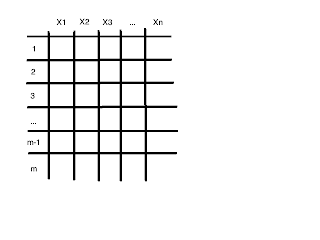We can randomly select from the entire data set a certain number of columns and rows and build a decision tree from them.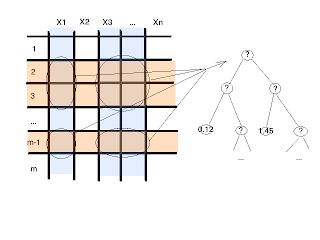Then we can repeat this process many times and get a lot of different trees. The process of the tree-building algorithm is very fast. And therefore, it will not be difficult for us to make as many trees as we need. At the same time, all of these trees are, in a sense, random, because we chose a random subset of data to create each of them.

The number of trees grown is often an important factor. This number may influence the achieved level of classification error. In addition, with sharply unbalanced classes (for example, a lot of 0 and only a small amount of 1), it is important to perform stratified sampling to even out the levels of classification error in each of these classes.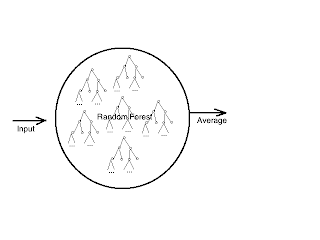In the original version of the algorithm, a random subset is selected at each step of the tree construction. But this does not change the essence and the results are comparable.

This surprisingly simple algorithm, the most difficult step in its implementation — the construction of the tree decision tree. And despite its simplicity, it gives very good results in real tasks. From a practical point of view, it has one huge advantage: it requires almost no configuration. If we take any other machine learning algorithm, be it regression or a neural network, they all have a bunch of parameters and you need to know what algorithms are better to apply for a specific task.

The random forest algorithm has essentially only one parameter: the size of the random subset selected at each step of the tree construction. This parameter is important, but even the default values provide very acceptable results.

# Random Forests vs Decision Trees

Both the random forest and decision trees are a type of classification algorithm, which are supervised in nature.

A decision tree is a graphical representation of all the possible solutions to a decision based on certain conditions. It’s called a decision tree because it starts with a single box (or root), which then branches off into a number of solutions, just like a tree.

Random forests involve building several decision trees based on sampling features and then making predictions based on majority voting among trees for classification problems or average for regression problems. This solves the problem of overfitting in Decision Trees.

When working with the forest, when constructing each tree at the stages of splitting the vertices, only a fixed number of randomly selected features of the training set is used (the second parameter of the method) and a complete tree is constructed (without truncation). In other words, each leaf of the tree contains observations of only one class.

# Random Forest Algorithm with Python and Scikit-LearnThe scikit-learn library has the following implementation of RF (below only for classification task):

```class sklearn.ensemble.RandomForestClassifier(n_estimators=10,
criterion=’gini’, max_depth=None, min_samples_split=2,
min_samples_leaf=1, min_weight_fraction_leaf=0.0,
max_features=’auto’, max_leaf_nodes=None, min_impurity_split=1e-07,
bootstrap=True, oob_score=False, n_jobs=1,
random_state=None, verbose=0, warm_start=False,
class_weight=None)```
They work with the algorithm according to the standard scheme adopted in scikit-learn:
```from sklearn.ensemble import RandomForestRegressor
from sklearn.metrics import roc_auc_score
# then — (X, y) -training, (X2, y2) — verification
# model — here (for contrast) consider the regressor
model = RandomForestRegressor(n_estimators=10 ,
oob_score=True,
random_state=1)
model.fit(X, y) # training
a = model.predict(X2) # prediction
print (“AUC-ROC (oob) = “, roc_auc_score(y, model.oob_prediction_))
print (“AUC-ROC (test) = “, roc_auc_score(y2, a))```

Let’s have a look at what the main parameters mean:

N_estimators
— Number of trees

The more trees, the better the quality, but the tuning and RF time also increase proportionally. Please note that often with an increase in n_estimators, the quality on the training sample increases (it can even reach 100%), and the quality on the test reaches the asymptote (you can estimate how many trees are enough for you).

Max_features
— The number of features to select for splitting is

As max_features increases, the time taken to build the forest increases, and the trees become “more uniform”. By default, it is sqrt (n) in classification problems and n / 3 in regression problems. This is the most important parameter! It is set up first of all (with a sufficient number of trees in the forest).

Min_samples_split
— The minimum number of objects at which splitting is performed

This parameter, as a rule, is not very important — you can leave the default value. The quality chart on the control may be similar to a “comb” (there is no clear optimum). As the parameter increases, the quality of training decreases, and the RF construction time decreases.

Min_samples_leaf
— Limit on the number of objects in leaves

Everything that has been described min_samples_split is also suitable for describing this parameter. Often you can leave the default value. By the way, it is usually recommended to use the value 5 in regression tasks (this is implemented in the randomForest library for R, and 1 in sklearn).

Max_depth
— Maximum tree depth

It is clear that the smaller the depth, the faster RF is built and works. With increasing depth, the quality of training sharply increases, but also in control, as a rule, it increases. It is recommended to use the maximum depth (except in cases where there are too many objects and very deep trees are obtained, the construction of which takes considerable time).

When using shallow trees, changing the parameters associated with limiting the number of objects in the sheet and for dividing does not lead to a significant effect (the leaves are “large” anyway). Shallow trees are recommended for use in problems with a large number of noise objects (emissions).

Criterion
— Cleavage criterion

In terms of meaning, this is a very important parameter, but in fact, there are no choices here. Two criteria are implemented in the sklearn library for regression: “mse” and “mae”, which correspond to the error functions that they minimize. Most tasks require using mse. For classification, the “gini” and “entropy” criteria are implemented, which correspond to the classical splitting criteria. A simple search will help you choose what to use in a particular task.

There is no sample size parameter in the sklearn implementation of a random forest, which regulates how many objects should be selected to build each tree. There is such a parameter in the R implementation, but, in fact, it is often optimal to choose from the entire sample. It is also recommended that you select a subsample with a return: bootstrap = True (this is bagging — bootstrap aggregating).

# Wrapping it up…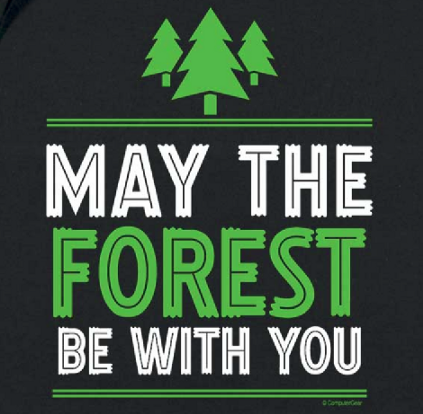So, random forest is a very easy to use algorithm. It has many advantages, and here are the most important points for me:

• random forests guarantee protection against overfitting even when the number of signs significantly exceeds the number of observations. By the way, you will not find such a function among other algorithms.
• building random forests is very simple — you only need two parameters that require minimal tuning
• random forests can be used not only for classification and regression tasks but also for the tasks of identifying the most informative features, clustering, highlighting anomalous observations and determining prototype classes

…………………………………….

Hope this post was useful and interesting for you! If you do anything cool with this information, leave a response in the comments below or reach out at any time on my
Instagram and Medium blog.

“Random Forests for Complete Beginners”
– Oleksii Kharkovyna

### Related Stories

Jan 06, 2023

#### Top 2 Online Data Science Courses to Improve your Career in 2023

The discipline of Data Science is expanding quickly and has enormous promise. It is used in various sectors, including manufacturing, retail, healt...By Nikos_DataSource
Nov 25, 2021

#### 5 Tips To Ace Your Job Interview For A Data Scientist Opening

5 Tips To Ace Your Job Interview For A Data Scientist Opening.PNG 795.94 KBImage SourceAspiring data scientists have a bright future ahead of them....By Daniel Morales
Nov 12, 2021

#### When to Avoid Deep Learning